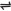## Tetraoxosulphate(VI) Acid, H2SO4, Preparation, Properties, and Uses

Tetraoxosulphate(VI) Acid is a chemical compound with the formula H2SO4.

Commercial Preparation of Tetraoxosulphate(VI) Acid - H2SO4

The industrial or commercial production of H2SO4 is through a process known as the contact process. The process involves:

1. The oxidation of sulphur(IV) oxide by air to sulphur(VI) oxide using a catalyst such as vanadium(V) oxide, V2O5.

 2SO2(g) + O22SO3(g)

SO2 is produced by burning sulphur or by burning pyrite, FeS2 in air.

2. The absorption of sulphur(VI) oxide, SO3 in conc. H2SO4 to form a fuming liquid called ‘Oleum’ - heptaoxosulphate(VI) acid

H2SO4(aq) + SO3(g) → H2S2O7(aq) - oleum

3. The oleum is diluted with correct amount of water to produce the conc. H2SO4.

H2S2O7(aq) + H2O(l) → 2H2SO4(aq)

Note: a direct absorption of SO3 in water is not done - the reaction is violently exothermic, and a mist of fine drops of dilute H2SO4 will fill the environment.

Properties of H2SO4

1. As an acid - H2SO4 is dibasic and ionizes almost completely in solution, this makes it a strong acid. Due to it being dibasic, it forms two kinds of salts with alkalis.

2NaOH(aq) + H2SO4(aq) → Na2SO4(aq) + 2H2O(l) and

NaOH(aq) + H2SO4(aq) → NaHSO4(aq) + H2O(l)

2. Action of dilute H2SO4 with metals

Reactive metals would displace hydrogen from dilute tetraoxosulphate(VI) acid.

Zn(s) + H2SO4(aq) → ZnSO4(aq) + H2(g)

Note:

* Less reactive metals such as copper will not displace hydrogen from dilute acid.

* Cold concentrated H2SO4 is not attacked by any metal in the complete absence of water.

3. Action on trioxocarbonate(IV)

Carbon(IV) oxide is liberated when H2SO4 is added onto a trioxocarbonate(IV).

Na2CO3(aq) + H2SO4(aq) → Na2SO4(aq) + H2O(l) + CO2(g)

On a piece of marble, which has the chemical formula CaCO3, the reaction obtained is prematurely stopped due to the formation of the sparingly soluble salt, CaSO4, which forms a deposit on the surface of the marble.

4. Conc. H2SO4 as an oxidizing agent

When hot and concentrated, the acid accepts electrons from reducing agents such as Cu or Zn.

It also oxidizes non-metals, such as carbon and sulphur, and is reduced to SO2 in the process.

S(s) + 2H2SO4(aq) → 2H2O(l) + 3SO2(g)

C(s) + 2H2SO4(aq) → 2H2O(l) + 2SO2(g) + CO2(g)

The SO2 given off is detected using a strip of filter paper moistened with potassium heptaoxodichromate(VI) solution which turns green - this is one of the tests for SO2 discussed earlier.

5. As a dehydrating agent

Tetraoxosulphate(VI) acid has a strong affinity for water. The addition of the acid to water (conc. acid should always be gradually added to water, with stirring of the liquid as the acid is added - to prevent the acid from forming a lower layer) produces a great amount of heat, hence the addition is not a physical change, but a chemical change.

H2SO4 is hygroscopic, that is, it absorbs water from the air and becomes dilute. When conc. H2SO4 is added to many compounds, it decomposes them by removing water molecules from them, that is, it dehydrates them.

An example is the removal of rust from iron, and the dehydration of sugar. The sugar becomes a black mass of carbon.

C12H22O11(s) + nH2SO4(l) → 12C(s) + (11H2O(l) + nH2SO4(aq))

In a similar way, conc. H2SO4 acts on the skin and cloth.

Test for Tetraoxosulphate(VI) Ion

The characteristic test for any soluble tetraoxosulphate(VI) is the formation of white precipitate (barium sulphate) when a solution of barium chloride, acidified with dilute HCl, or when a solution of barium nitrate, acidified with dilute nitric acid is reacted with it.

BaCl2(aq) + H2SO4(aq) → 2HCl(aq) + BaSO4(s)

Ba(NO3)2(aq) + H2SO4(aq) → 2HNO3(aq) + BaSO4(s) - white precipitate

Uses of H2SO4

Below are some of the uses of H2SO4

1. For the manufacture of fertilizer, such as ammonium tetraoxosulphate(VI), NH4SO4, and superphosphates.

2. For the manufacture of paints and pigments.

3. For the manufacture of artificial and natural fibre.

4. For the manufacture of metallic tetraoxosulphate(VI), HCl, HNO3, HF, and plastics.

5. For the manufacture of detergents, dyes, explosives and drugs.

6. For the extraction of metals, example, in pickling (cleaning) iron and steel before plating them with tin or zinc.

7. As electrolyte in lead-acid storage battery.

8. In petroleum refining, where to is used to wash impurities out of gasoline and other refinery products.